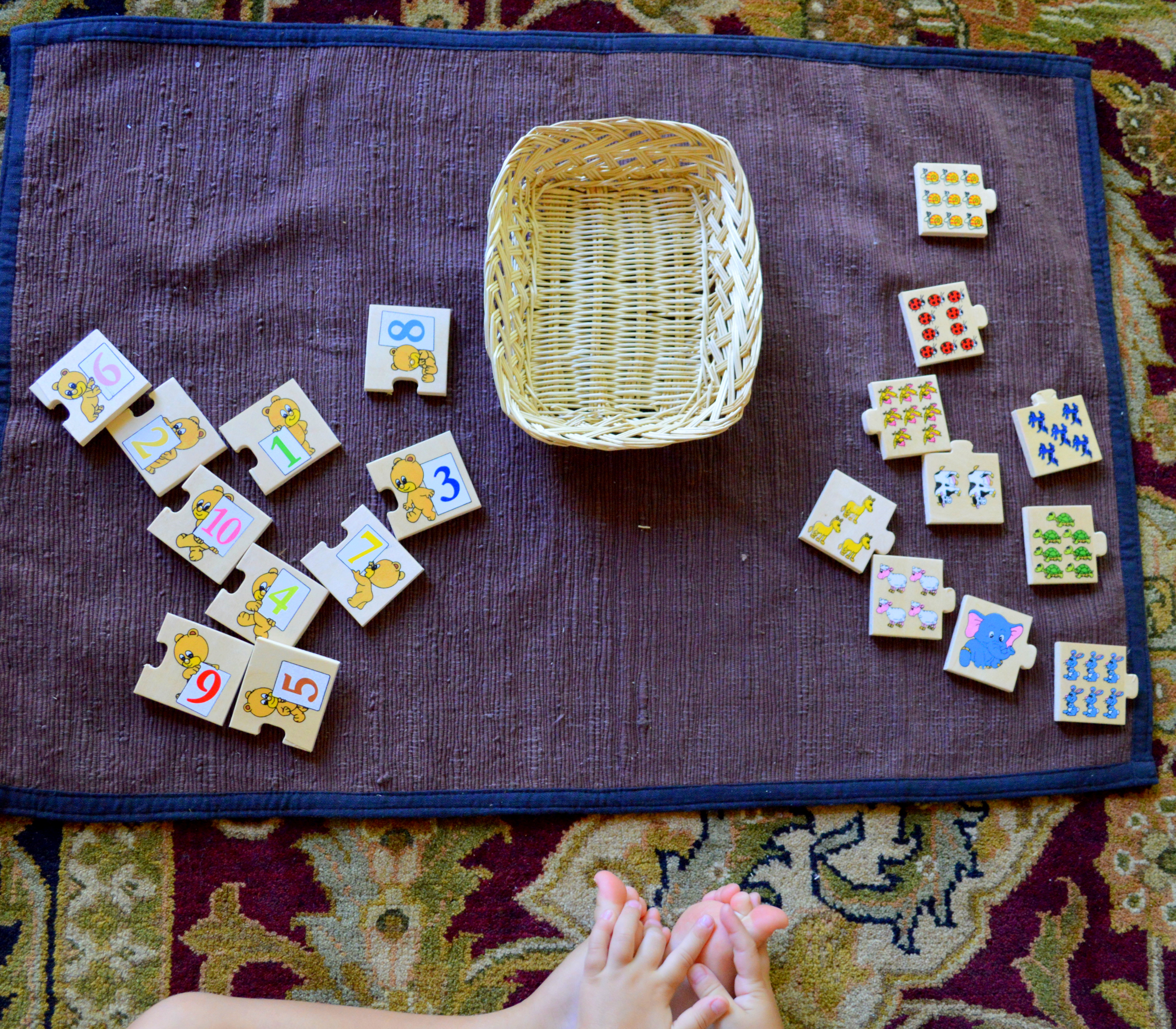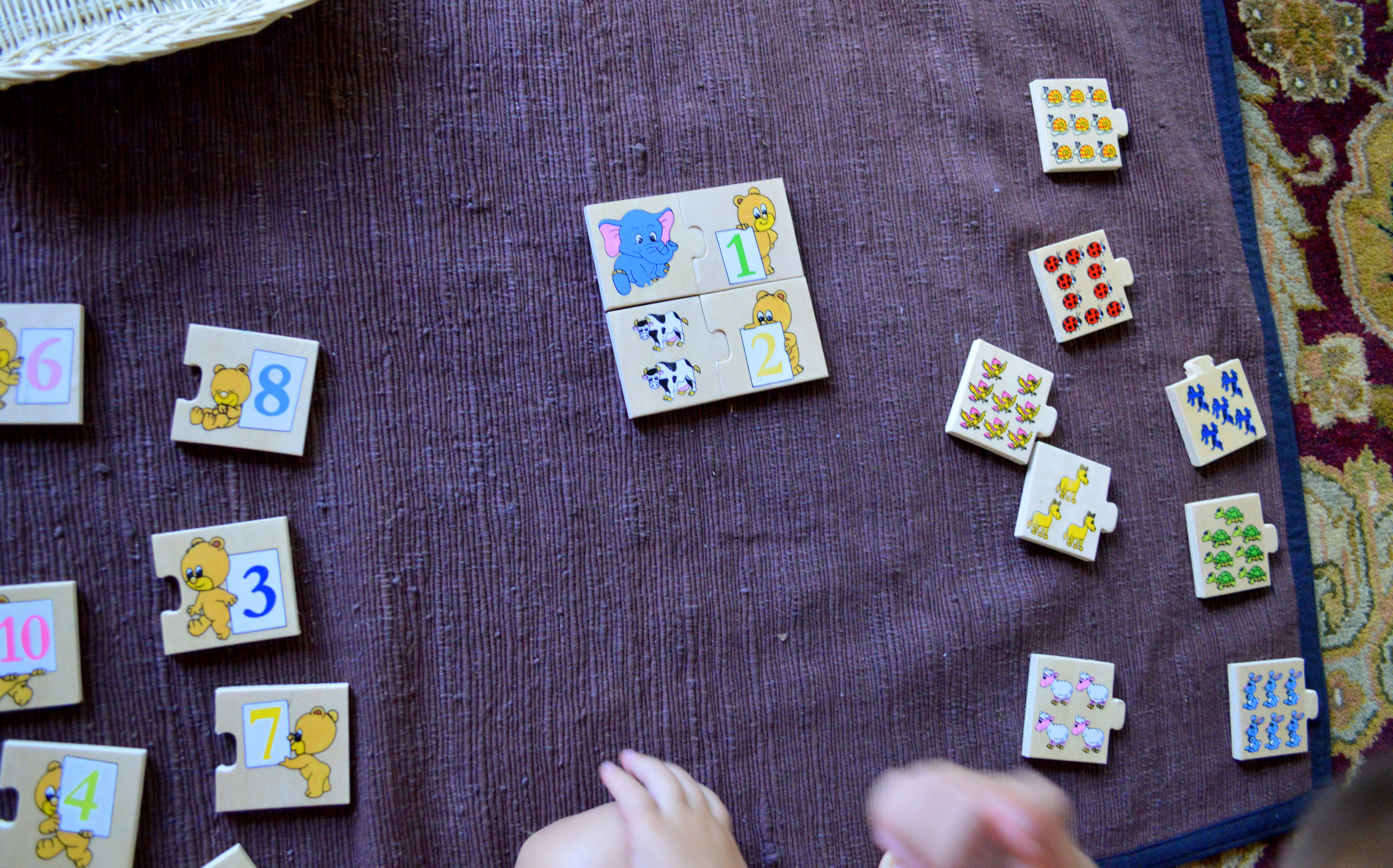This wooden number-to-quantity interlocking self-correcting Numbers Puzzle (buy similar here) is more abstract as a toddler is not actually holding any quantity to substantiate the value (like with Spindles or Numbers Rods), but rather matches a numeral (e.g. number 2) to the quantity shown on a picture (2 cows). This puzzle is great for matching and counting skills since the pictures are colorful and feature familiar objects/animals.A child would first sort the puzzle pieces into two piles: one with numbers and the other with quantities (pictures of the objects).Adrian then would sequence numbers in order from 1-10, by counting objects on a picture and matching them to the correct numeral.

To substantiate the concept of numeral vs quantity, you may want to present a 3🅿️🌠 Three Period Lesson. (Read a detailed post about the presentation here).

At 34 months, Adrian is able to correctly complete the entire 3🅿️🌠 Three Period Lesson with numerals one through ten.

Presenting a 3🅿️🌠 Three Period Lesson:

1. (P1) “This is 1, this is 2, ….3”
2. (P2) “Will you show me 1? Will you show me 2? … 3? "
3. (P3) “What is this?"Show me 5"; "Where is 9?"; "What number is this?" Adrian would correctly answer each time.

Thus, at 34 months, when asked randomly, Adrian can orderly sequence, recognize and enunciate each number from 1-10. I believe that such understanding was only achieved through the concrete representation of Montessori math materials, which necessarily build on each other just like in other areas. So, before introducing this puzzle, you would start with small concept of numbers one through 10. For example, number "one" is represented by a numeral -1 as well as by quantity when a child is actually holding one apple/car/spindle in his/her hands. Such association of quantity to numeral is very concrete in nature: a child is holding one, two, or three spindles and realizes how differently it feels than holding ten. Such helps children understand in a concrete way basic mathematical concepts, while instilling the love for learning, numbers, and math.